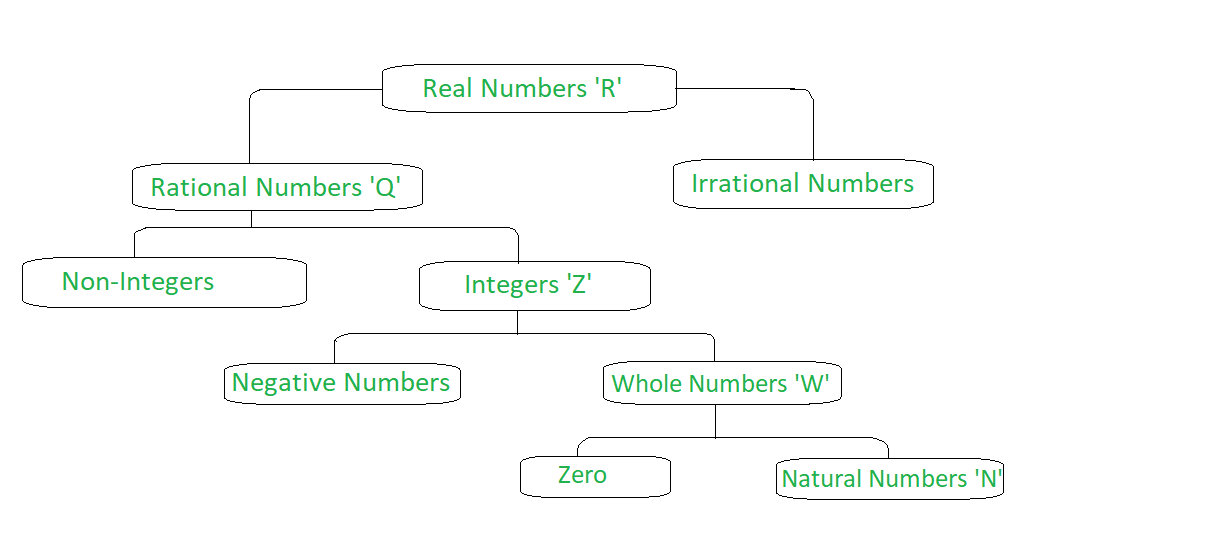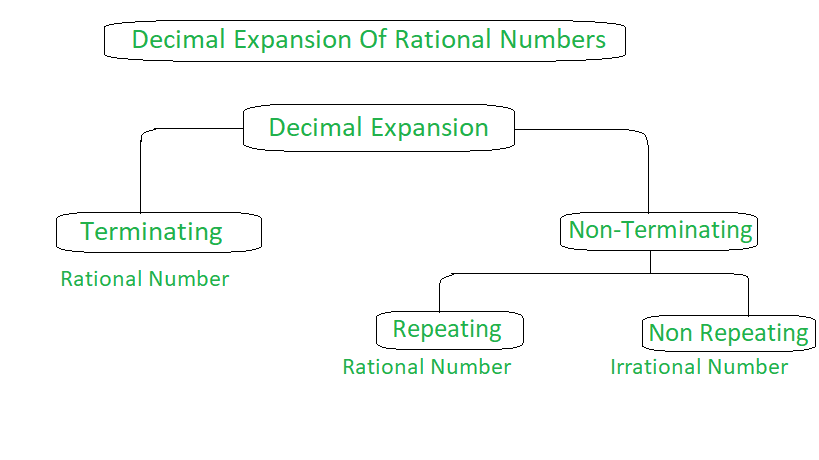Related Articles

# Decimal Expansion of Real Numbers

• Last Updated : 19 Jan, 2021

The Combination of a set of rational and irrational numbers is called real numbers. All the real numbers can be expressed on the number line. The numbers other than real numbers that cannot be represented on the number line are called imaginary numbers (unreal numbers). They are used to represent complex numbers. Below is a classification diagram of real numbers.## Decimal Expansion

Before going into a representation of the decimal expansion of rational numbers, let us understand what rational numbers are. A Rational Number is a number that can be written in form of p/q  where p, q are Integers and q != 0. Example: 2/3, 1/4, 4/5, etc. Rational numbers are denoted by Q. As every Integer can be represented in p/q form so all Integers are rational numbers.

Example: -1, -2, -6, 4, 5 can be represented as -1/1, -2/1, 4/1, 5/1

There are generally 3 types of decimal expansion:

1. Terminating
2. Non-terminating Repeating
3. Non-terminating Non Repeating### Terminating Decimals

Terminating decimals are those decimal numbers that have a finite number of digits. That means the number comes to end after a decimal point after a certain number of repetitions.

Example: 0.5, 0.678, 14.123445, 1.23, 0.00024, etc.

### Non-Terminating Decimals

Non-Terminating decimals are those decimal numbers that have an infinite number of digits. Here the number doesn’t comes to end.

Example: 1.33333….., 52.36363636…, 2.343537684904…, 3.1415926535897…, etc.

Repeating Decimals: Repeating decimals are those numbers in which a specific number repeats uniformly after a decimal point.

Example: 0.5555…, 13.262626…, 1.8769876…, etc.

Note: Non terminating and repeating decimals are rational numbers. They can be expressed in p/q form, where q != 0.

Non Repeating Decimals: In Non Repeating decimals, there is no uniform repetition of a number.

Example: 4.345627238…, 1.61803398…, 2.718281828459.., 1342.352567545…, etc.

Note: Non terminating and nonrepeating decimals are irrational numbers. They cannot be expressed in p/q form.

## Converting Repeating Decimals to Fractions

### Case 1: Fractions of type  0.yyyyy… or 0.xyxyxyxy…… or 0.xyzxyzxyz…. etc

Example 1: Convert 0.4444…. to a fraction?

Solution:

Let x = 0.4444…. — eq(1)

as only one term(4) is repeating multiply eq(1) with 10.

10x = 4.4444…. — eq(2)

Now substract eq(1) from eq(2) [eq(2) – eq(1)]

9x = 4

=> x = 4/9

Example 2: Convert 0.45454545…. to a fraction?

Solution:

Formula = Repeated term/number of 9’s for repeated term

0.45454545… = 45 (repeated term)/99 (Two 9’s as only two terms are repeating) = 45/99

### Case 2: Fractions of the form 0.abcxyxyxyxyxy… (the combination of repeating and nonrepeating)

Formula: 0.abcxyxyxyxy…. = abcxy – (xy/number of 9’s for repeating term and number of 0’s for nonrepeating term)

Example 1: Convert 0.45232323… to fraction?

Solution:

Here 45 is nonrepeating and 23 is repeating. So in the numerator, we subtract the nonrepeating term(45) from the number and as we have two terms as repeating(2, 3). In the denominator, we place two 9’s followed by two zeros, as we have two non-repeating terms(45).

0.45232323… = 4523 – (45/9900)

Example 2: Convert 0.000456456… to fraction?

Solution:

Here 000 is nonrepeating and 456 is repeating. So in Numerator, we subtract 000 from 000456. In the denominator, as we have three repeating terms we place three 9’s followed by three 0’s for three non-repeating terms.

0.000456456…. = 000456 – (000/999000)

= 456/999000

### Properties Of Rational Numbers

Closure Property

If we add, subtract or multiply two rational numbers then the result is a rational number. Closure property is not applicable for the division as any number divided by zero(rational number) is undefined. Other than zero it’s applicable.

Example:

2/3 + 3/4 = 17/12

3/4 – 2/6 = 5/12

4/5 * 3/2 = 12/10

Commutative Property

For any two rational numbers, addition and multiplication are commutative whereas division and subtraction don’t follow the commutative property.

Commutative law of addition: a + b = b + a

Example: 1/2 + 4/3 = 4/3 + 1/2 = 11/6

Commutative law of Multiplication: a * b = b * a

Example: 2/7 * 5/8 = 5/8 * 2/7 = 10/56

Associative Property

For addition and multiplication, Associative Property is followed by rational numbers. Let a, b, c be three rational numbers then,

Associative property of addition: a + (b + c) = (a + b) + c

Associative property of multiplication: a * (b * c) = (a * b) * c

Distributive Property

According to Distributive property let a, b, c be three rational numbers then a * (b + c) = a * b + a * c.

Example:

1/2 * (2/3 + 1/3) = 3/6

=> 1/2 * 2/3 + 1/2 * 1/3 = 3/6

Identity Property

Additive Identity of Rational numbers is 0

Example: 5/9 + 0 = 5/9

Multiplicative Identity of Rational numbers is 1

Example: 7/8 * 1 = 7/8

Inverse Property

Additive Inverse of a Rational Number  a/b is -a/b

Example: 4/6 additive inverse is -4/6

Multiplicative Inverse of a Rational Number a/b is b/a

Example: 3/8 multiplicative inverse is 8/3

Attention reader! Don’t stop learning now. Join the First-Step-to-DSA Course for Class 9 to 12 students , specifically designed to introduce data structures and algorithms to the class 9 to 12 students

My Personal Notes arrow_drop_up